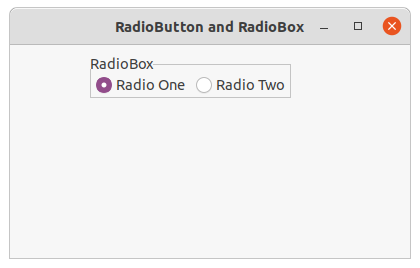Skip to content
Related Articles
wxPython – GetRowCount() function in wx.RadioBox
• Last Updated : 30 Jun, 2020

In this article we are going to learn about GetRowCount() method associated with wx.RadioBox class of wxPython. GetRowCount() method is a simple method and used in order to return the number of item rows in the radiobox.

No parameters are required by GetRowCount() function.

Syntax: wx.RadioBox.GetRowCount(self)

Parameters No parameters are required by GetRowCount() function.

Return Type:
int

Code Example:

 `import` `wx`` ` ` ` `class` `FrameUI(wx.Frame):`` ` `    ``def` `__init__(``self``, parent, title):``        ``super``(FrameUI, ``self``).__init__(parent, title ``=` `title, size ``=``(``300``, ``200``))`` ` `        ``# function for in-frame components``        ``self``.InitUI()`` ` `    ``def` `InitUI(``self``):``        ``# parent panel for radio box``        ``pnl ``=` `wx.Panel(``self``)`` ` `        ``# list of choices``        ``lblList ``=` `[``'Radio One'``, ``'Radio Two'``]`` ` `        ``# create radio boc containing above list``        ``self``.rbox ``=` `wx.RadioBox(pnl, label ``=``'RadioBox'``, pos ``=``(``80``, ``10``), choices ``=` `lblList,``                                          ``majorDimension ``=` `1``, style ``=` `wx.RA_SPECIFY_COLS)`` ` `        ``# set tooltip for first tool``        ``self``.rbox.SetItemToolTip(``0``, ``"Item One"``)`` ` `        ``# print the total rows of item inside radio box``        ``print` `(``self``.rbox.GetRowCount())`` ` ` ` `        ``# set frame in centre``        ``self``.Centre()``        ``# set size of frame``        ``self``.SetSize((``400``, ``250``))``        ``# show output frame``        ``self``.Show(``True``)`` ` ` ` `# wx App instance``ex ``=` `wx.App()``# Example instance``FrameUI(``None``, ``'RadioButton and RadioBox'``)``ex.MainLoop()`

Console Output:

```1
```

Output Window:Attention geek! Strengthen your foundations with the Python Programming Foundation Course and learn the basics.

To begin with, your interview preparations Enhance your Data Structures concepts with the Python DS Course. And to begin with your Machine Learning Journey, join the Machine Learning – Basic Level Course

My Personal Notes arrow_drop_up